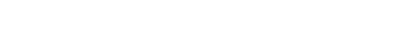#### Revision Notes

##### What is a quadratic equation?

A quadratic equation looks like (or can/should be made to look like) this:

ax2 + bx + c = 0    (as long as a ≠ 0)

##### What is the quadratic formula?

The Quadratic Formula is a way of finding the roots (also called solutions) of a quadratic equation (if they exist – see Exam Tip 1):##### A quick note about calculators

If you have an “advanced” scientific calculator (like ones from the Casio Classwiz range) you may find it will solve quadratic equations for you. However, at GCSE, you should avoid using this feature as marks are awarded for method. At best you can use them to check your final answers but be aware that such calculators often show solutions even when the roots are not real.

##### When do you use the quadratic formula?

You can use the Quadratic Formula whenever you need to solve a quadratic equation UNLESS you are specifically asked to do it by Factorising  or Completing the Square. (You could still use the formula to check your answer!)

##### How do you use the quadratic formula?

The safest way to avoid errors is to break the formula down into stages:

1. WRITE DOWN the values of a, b and c – ie  a = … , b = … and c = …
2. Work out the DISCRIMINANT, d = b2 – 4ac
3. Substitute values into the QUADRATIC FORMULA including d
4. Work out the TWO ANSWERS on your calculator

(Type in the formula twice, once with the “+” and once with the “-”)

#### Example#### Question#### Answer## Want to aim for a Level 9?

See if you’ve got what it takes. Test yourself with our topic questions.### Author: Simon

After 24 years teaching A Level Further Maths, Maths whizz Simon focused his attention on tutoring. He’s helped thousands of young people make sense of Maths and now he’s helping you too – aren’t you lucky! He’s even worked as an Edexcel examiner, so knows exactly what they’re looking for.